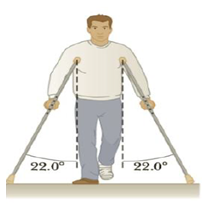Chapter 4, Problem 49P

Chapter
Section
Textbook Problem

The person in Figure P4.49 weighs 170. lb. Each crutch makes an angle of 22.0° with the vertical (as seen from the front). Half of the person’s weight is supported by the crutches, the other half by the vertical forces exerted by the ground on his feet. Assuming he is at rest and the force exerted by the ground on the crutches acts along the crutches, determine (a) the smallest possible coefficient of friction between crutches and ground and (b) the magnitude of the compression force supported by each crutch.Figure P4.49

(a)

To determine
The smallest possible value of co-efficient of friction.

Explanation

Given Info: Weight of the person is 170 lb.

The free body diagrams are given below.

From the free body diagram of the person,

F1sin22ο=F2sin22ο (I)

Nf+F1cos22ο+F2cos22ο=mg (II)

• Fs is the frictional force.
• F1 and F2 are the forces along the two crutches.
• g is the acceleration due to gravity.
• m is the mass of the person.
• Nf is the normal force corresponding to the feet of the person.
• Nt is the normal force corresponding to the tip of the crutch.

From Equation (II),

F1=F2

Let F1 = F2 =F

Substitute F for F1 and F2 in Equation (II).

Nf+2Fcos22°=W (III)

Since, vertical forces support half of the person’s weight, the normal force is,

Nf=W2

Substitute W/2 for Nf in Equation (III) and re-arrange to get F

(b)

To determine
The magnitude of the compression force.

Still sussing out bartleby?

Check out a sample textbook solution.

See a sample solution

The Solution to Your Study Problems

Bartleby provides explanations to thousands of textbook problems written by our experts, many with advanced degrees!

Get Started

Find more solutions based on key concepts